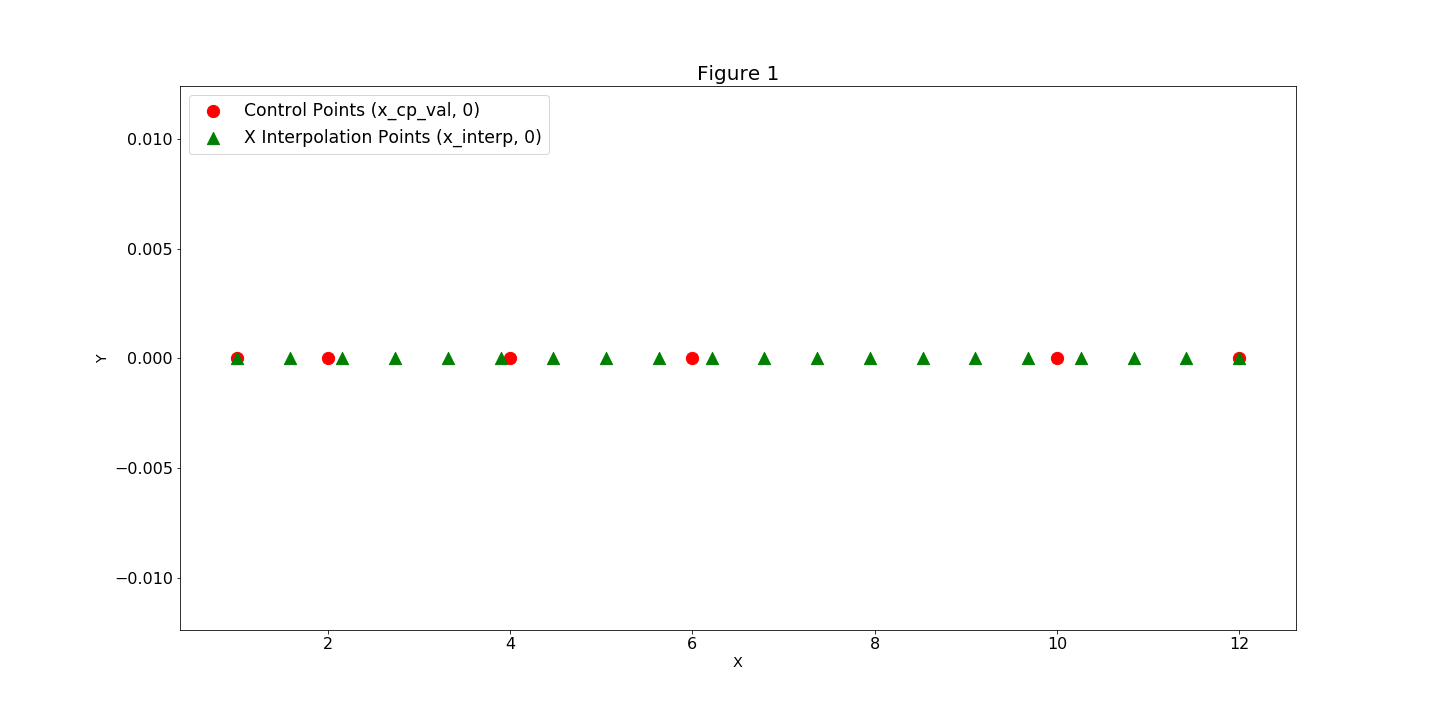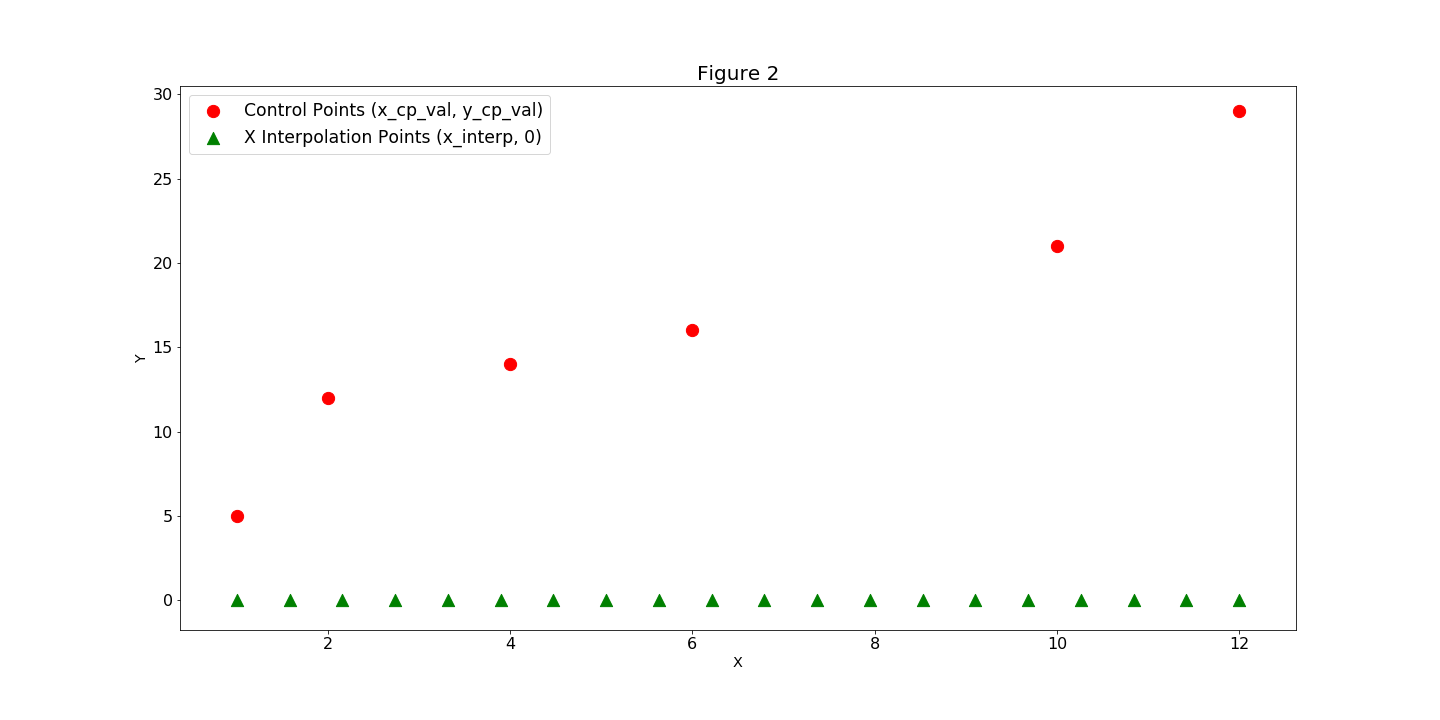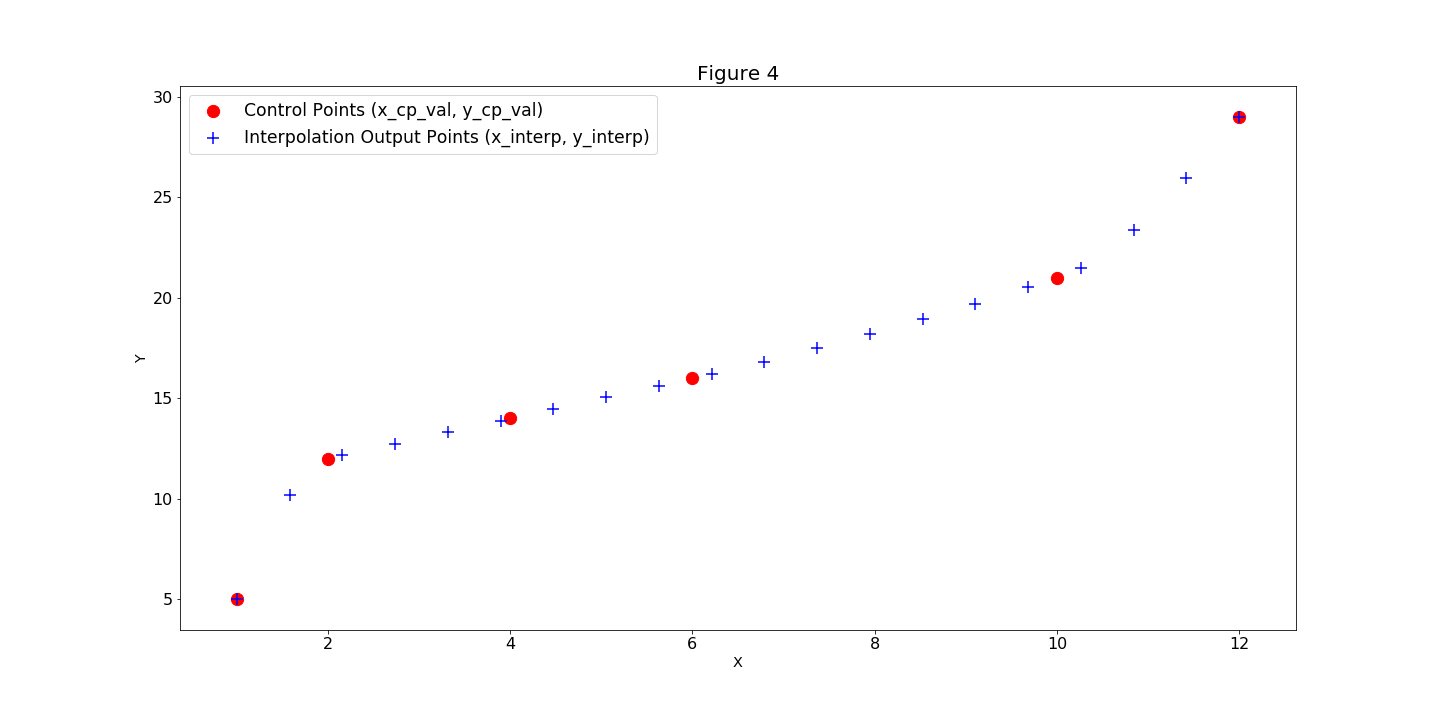# SplineComp#

SplineComp allows you to represent a larger dimensional variable with a smaller dimensional variable by using an interpolation algorithm. This is useful for reducing the size of an optimization problem by decreasing the number of design variables it solves. The spatial distribution of the points, in both the original and interpolated spaces is typically uniform but other distributions can be used.

Note

OpenMDAO contains two components that perform interpolation: SplineComp and MetaModelStructuredComp. While they provide access to mostly the same algorithms, their usage is subtly different. The fundamental differences between them are as follows:

MetaModelStructuredComp is used when you have a set of known data values y on a structured grid x and want to interpolate a new y value at a new x location that lies inside the grid. In this case, you generally start with a known set of fixed “training” values and their locations.

SplineComp is used when you want to create a smooth curve with a large number of points, but you want to control the shape of the curve with a small number of control points. The x locations of the interpolated points (and where applicable, the control points) are fixed and known, but the y values at the control points vary as the curve shape is modified by an upstream connection.

MetaModelStructuredComp can be used for multi-dimensional design spaces, whereas SplineComp is restricted to one dimension.

The following methods are available by setting the ‘method’ option:

Method

Order

Description

slinear

1

Basic linear interpolation

lagrange2

2

Second order Lagrange polynomial

lagrange3

3

Third order Lagrange polynomial

akima

3

Interpolation using Akima splines

cubic

3

Cubic spline, with continuity of derivatives between segments

bsplines

var.

BSplines, default order is 4.

scipy_slinear

1

Scipy linear interpolation. Same as slinear, though slower

scipy_cubic

3

Scipy cubic interpolation. More accurate than cubic, but slower

scipy_quintic

5

Scipy quintic interpolation. Most accurate, but slowest

## SplineComp Options#

OptionDefaultAcceptable ValuesAcceptable TypesDescription
always_optFalse[True, False]['bool']If True, force this component to be included in the optimization loop if the Problem option "group_by_pre_opt_post" is True.
distributedFalse[True, False]['bool']If True, set all variables in this component as distributed across multiple processes
interp_options{}N/A['dict']Dict contains the name and value of options specific to the chosen interpolation method.
methodakima['slinear', 'lagrange2', 'lagrange3', 'cubic', 'akima', 'bsplines', 'scipy_cubic', 'scipy_slinear', 'scipy_quintic']N/ASpline interpolation method to use for all outputs.
num_cpN/AN/A['int']Number of spline control points. Optional alternative to x_cp_val. Required for bsplines. If None, num_cp will be a linspace from 0 to 1.
run_root_onlyFalse[True, False]['bool']If True, call compute, compute_partials, linearize, apply_linear, apply_nonlinear, and compute_jacvec_product only on rank 0 and broadcast the results to the other ranks.
vec_size1N/A['int']Number of points to evaluate at once.
x_cp_valN/AN/A['list', 'ndarray']List/array of x control point values, must be monotonically increasing. Not applicable for bsplines.
x_interp_val**Required**N/A['list', 'ndarray']List/array of x interpolated point values.

## SplineComp Constructor#

The call signature for the SplineComp constructor is:

SplineComp.__init__(**kwargs)[source]

Initialize all attributes.

## SplineComp Basic Example#

In our example, we have a pre-generated curve that is described by a series of values y_cp at a sequence of locations x_cp, and we would like to interpolate new values at multiple locations between these points. We call these new fixed locations at which to interpolate: x. When we instantiate a SplineComp, we specify these x_cp and x locations as numpy arrays and pass them in as constructor keyword arguments. (Figure 1). Next we’ll add our y_cp data in by calling the add_spline method and passing the y_cp values in as the keyword argument y_cp_val (Figure 2). SplineComp computes and outputs the y_interp values (Figure 3).import numpy as np
import openmdao.api as om

xcp = np.array([1.0, 2.0, 4.0, 6.0, 10.0, 12.0])
ycp = np.array([5.0, 12.0, 14.0, 16.0, 21.0, 29.0])
n = 50
x = np.linspace(1.0, 12.0, n)

prob = om.Problem()

akima_option = {'delta_x': 0.1}
comp = om.SplineComp(method='akima', x_cp_val=xcp, x_interp_val=x,
interp_options=akima_option)

prob.setup(force_alloc_complex=True)
prob.run_model()

print(prob.get_val('akima1.y_val'))

[[ 5.          7.20902005  9.21276849 10.81097162 11.80335574 12.1278001
12.35869145 12.58588536 12.81022332 13.03254681 13.25369732 13.47451633
13.69584534 13.91852582 14.14281484 14.36710105 14.59128625 14.81544619
15.03965664 15.26399335 15.48853209 15.7133486  15.93851866 16.16573502
16.39927111 16.63928669 16.8857123  17.1384785  17.39751585 17.66275489
17.93412619 18.21156029 18.49498776 18.78433915 19.07954501 19.38053589
19.68724235 19.99959495 20.31752423 20.64096076 20.96983509 21.37579297
21.94811407 22.66809748 23.51629844 24.47327219 25.51957398 26.63575905
27.80238264 29.        ]]


## SplineComp Multiple Splines#

SplineComp supports multiple splines on a fixed x_interp grid. Below is an example of how a user can setup two splines on a fixed grid. To do this the user needs to pass in names to give to the component input and output. The initial values for y_cp can also be specified here.

x_cp = np.array([1.0, 2.0, 4.0, 6.0, 10.0, 12.0])
y_cp = np.array([5.0, 12.0, 14.0, 16.0, 21.0, 29.0])
y_cp2 = np.array([1.0, 5.0, 7.0, 8.0, 13.0, 16.0])
n = 50
x = np.linspace(1.0, 12.0, n)

prob = om.Problem()

comp = om.SplineComp(method='akima', x_cp_val=x_cp, x_interp_val=x)

prob.setup(force_alloc_complex=True)
prob.run_model()


## Specifying Options for ‘akima’#

When you are using the ‘akima’ method, there are two akima-specific options that can be passed in to the SplineComp constructor. The ‘delta_x’ option is used to define the radius of the smoothing interval that is used in the absolute values functions in the akima calculation in order to make their derivatives continuous. This is set to zero by default, which effectively turns off the smoothing. The ‘eps’ option is used to define the value that triggers a division-by-zero safeguard; its default value is 1e-30.

x_cp = np.array([1.0, 2.0, 4.0, 6.0, 10.0, 12.0])
y_cp = np.array([5.0, 12.0, 14.0, 16.0, 21.0, 29.0])

n = 50
x = np.linspace(1.0, 12.0, n)

prob = om.Problem()
model = prob.model

# Set options specific to akima
akima_option = {'delta_x': 0.1, 'eps': 1e-30}

comp = om.SplineComp(method='akima', x_cp_val=x_cp, x_interp_val=x,
interp_options=akima_option)

prob.setup(force_alloc_complex=True)
prob.run_model()


## Specifying Options for ‘bsplines’#

When you use the ‘bsplines’ method, you can specify the bspline order by defining ‘order’ in an otherwise empty dictionary and passing it in as ‘interp_options’.

In addition, when using ‘bsplines’, you cannot specify the ‘x_cp’ locations because the bspline formulation differs from other polynomial interpolants. When using bsplines, you should instead specify the number of control points using the ‘num_cp’ argument.

prob = om.Problem()
model = prob.model

n_cp = 80
n_point = 160

t = np.linspace(0, 3.0*np.pi, n_cp)
tt = np.linspace(0, 3.0*np.pi, n_point)
x = np.sin(t)

# Set options specific to bsplines
bspline_options = {'order': 3}

comp = om.SplineComp(method='bsplines', x_interp_val=tt, num_cp=n_cp,
interp_options=bspline_options)

prob.setup(force_alloc_complex=True)
prob.set_val('x', x)
prob.run_model()


## SplineComp Interpolation Distribution#

The cell_centered function takes the number of cells, and the start and end values, and returns a vector of points that lie at the center of those cells. The ‘node_centered’ function reproduces the functionality of numpy’s linspace. Finally, the sine_distribution function creates a sinusoidal distribution, in which points are clustered towards the ends. A ‘phase’ argument is also included, and a phase of pi/2.0 clusters the points in the center with fewer points on the ends.

Note

We have included three different distribution functions for users to replicate functionality that used to be built-in to the individual akima and bsplines components.

openmdao.utils.spline_distributions.sine_distribution(num_points, start=0.0, end=1.0, phase=3.141592653589793)[source]

Sine distribution of control points.

Parameters:
num_pointsint

Number of points to predict at.

startint or float

Minimum value to interpolate at.

endint or float

Maximum value to interpolate at.

phasefloat

Phase of the sine wave.

Returns:
ndarray

Values to interpolate at.

openmdao.utils.spline_distributions.cell_centered(num_cells, start=0.0, end=1.0)[source]

Cell centered distribution of control points.

Parameters:
num_cellsint

Number of cells.

startint or float

Minimum value to interpolate at.

endint or float

Maximum value to interpolate at.

Returns:
ndarray

Values to interpolate at.

openmdao.utils.spline_distributions.node_centered(num_points, start=0.0, end=1.0)[source]

Distribute control points.

Parameters:
num_pointsint

Number of points to predict.

startint or float

Minimum value to interpolate at.

endint or float

Maximum value to interpolate at.

Returns:
ndarray

Values to interpolate at.

Below is an example of sine_distribution

from openmdao.utils.spline_distributions import sine_distribution

x_cp = np.linspace(0., 1., 6)
y_cp = np.array([5.0, 12.0, 14.0, 16.0, 21.0, 29.0])
n = 20
x = om.sine_distribution(20, start=0, end=1, phase=np.pi)

prob = om.Problem()

comp = om.SplineComp(method='akima', x_cp_val=x_cp, x_interp_val=x)

prob.setup(force_alloc_complex=True)
prob.run_model()

print(prob.get_val('akima1.y_val'))

[[ 5.          5.32381994  6.28062691  7.79410646  9.64169506 11.35166363
12.26525921 12.99152288 13.77257256 14.58710327 15.41289673 16.28341046
17.96032258 20.14140712 22.31181718 24.40891577 26.27368825 27.74068235
28.67782484 29.        ]]


## Standalone Interface for Spline Evaluation#

The underlying interpolation algorithms can be used standalone (i.e., outside of the SplineComp) through the InterpND class. This can be useful for inclusion in another component. The following example shows how to create and evaluate a standalone Akima spline:

from openmdao.components.interp_util.interp import InterpND

xcp = np.array([1.0, 2.0, 4.0, 6.0, 10.0, 12.0])
ycp = np.array([5.0, 12.0, 14.0, 16.0, 21.0, 29.0])
n = 50
x = np.linspace(1.0, 12.0, n)

interp = InterpND(method='akima', points=xcp, x_interp=x, delta_x=0.1)
y = interp.evaluate_spline(ycp)

print(y)

[ 5.          7.20902005  9.21276849 10.81097162 11.80335574 12.1278001
12.35869145 12.58588536 12.81022332 13.03254681 13.25369732 13.47451633
13.69584534 13.91852582 14.14281484 14.36710105 14.59128625 14.81544619
15.03965664 15.26399335 15.48853209 15.7133486  15.93851866 16.16573502
16.39927111 16.63928669 16.8857123  17.1384785  17.39751585 17.66275489
17.93412619 18.21156029 18.49498776 18.78433915 19.07954501 19.38053589
19.68724235 19.99959495 20.31752423 20.64096076 20.96983509 21.37579297
21.94811407 22.66809748 23.51629844 24.47327219 25.51957398 26.63575905
27.80238264 29.        ]


Similiarly, the following example shows how to create a bspline:

from openmdao.components.interp_util.interp import InterpND

xcp = np.array([1.0, 2.0, 4.0, 6.0, 10.0, 12.0])
ycp = np.array([5.0, 12.0, 14.0, 16.0, 21.0, 29.0])
n = 50
x = np.linspace(1.0, 12.0, n)

interp = InterpND(method='bsplines', num_cp=6, x_interp=x)

y = interp.evaluate_spline(ycp)

print(y)

[ 9.21614583  9.90911525 10.52244151 11.06231159 11.53491244 11.94643105
12.30305438 12.61096939 12.87636305 13.10542234 13.30433422 13.47928566
13.63646363 13.7820551  13.92203064 14.05954727 14.19579437 14.33192094
14.46907599 14.60840854 14.75106758 14.89820214 15.05096121 15.2104938
15.37794893 15.5544756  15.74122282 15.9393396  16.14997495 16.37427787
16.61339737 16.86848247 17.14102103 17.43486416 17.75486932 18.10589772
18.49281052 18.92046894 19.39373414 19.91746734 20.4965297  21.13578243
21.8400867  22.61430372 23.46329467 24.39192074 25.40504312 26.507523
27.70422156 29.        ]


You can also compute the derivative of the interpolated output with respect to the control point values by setting the “compute_derivate” argument to True:

from openmdao.components.interp_util.interp import InterpND

xcp = np.array([1.0, 2.0, 4.0, 6.0, 10.0, 12.0])
ycp = np.array([5.0, 12.0, 14.0, 16.0, 21.0, 29.0])
n = 5
x = np.linspace(1.0, 12.0, n)

interp = InterpND(method='akima', points=xcp, x_interp=x, delta_x=0.1)
y, dy_dycp = interp.evaluate_spline(ycp, compute_derivative=True)

print(dy_dycp)

[[ 1.00000000e+00  0.00000000e+00  0.00000000e+00  0.00000000e+00
0.00000000e+00  0.00000000e+00]
[-1.86761492e-06  3.31278014e-02  1.05874907e+00 -9.18750000e-02
0.00000000e+00  0.00000000e+00]
[ 0.00000000e+00  0.00000000e+00 -2.10964627e-01  1.19119941e+00
2.02602810e-02 -4.95062934e-04]
[ 0.00000000e+00  0.00000000e+00 -2.64126732e-01  5.82784977e-01
6.83151998e-01 -1.81024253e-03]
[ 0.00000000e+00  0.00000000e+00  0.00000000e+00  0.00000000e+00
0.00000000e+00  1.00000000e+00]]